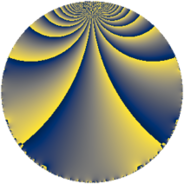# Properties

 Label 63.3.kLevel $63$ Weight $3$ Character orbit 63.k Rep. character $\chi_{63}(31,\cdot)$ Character field $\Q(\zeta_{6})$ Dimension $28$ Newform subspaces $1$ Sturm bound $24$ Trace bound $0$

# Related objects

## Defining parameters

 Level: $$N$$ $$=$$ $$63 = 3^{2} \cdot 7$$ Weight: $$k$$ $$=$$ $$3$$ Character orbit: $$[\chi]$$ $$=$$ 63.k (of order $$6$$ and degree $$2$$) Character conductor: $$\operatorname{cond}(\chi)$$ $$=$$ $$63$$ Character field: $$\Q(\zeta_{6})$$ Newform subspaces: $$1$$ Sturm bound: $$24$$ Trace bound: $$0$$

## Dimensions

The following table gives the dimensions of various subspaces of $$M_{3}(63, [\chi])$$.

Total New Old
Modular forms 36 36 0
Cusp forms 28 28 0
Eisenstein series 8 8 0

## Trace form

 $$28 q + q^{2} - 3 q^{3} - 23 q^{4} + 12 q^{6} - 16 q^{8} + 9 q^{9} + O(q^{10})$$ $$28 q + q^{2} - 3 q^{3} - 23 q^{4} + 12 q^{6} - 16 q^{8} + 9 q^{9} - 6 q^{10} - 14 q^{11} - 3 q^{12} + 15 q^{13} - 11 q^{14} - 18 q^{15} - 27 q^{16} - 33 q^{17} + 33 q^{18} - 6 q^{19} + 108 q^{20} + 12 q^{21} - 10 q^{22} - 68 q^{23} + 42 q^{24} - 62 q^{25} + 54 q^{26} - 81 q^{27} - 16 q^{28} + 70 q^{29} - 6 q^{30} + 45 q^{31} + 153 q^{32} - 114 q^{33} + 12 q^{34} + 18 q^{35} - 174 q^{36} + 9 q^{37} - 120 q^{39} - 234 q^{41} - 51 q^{42} + 30 q^{43} + 51 q^{44} + 276 q^{45} - 22 q^{46} - 111 q^{47} + 147 q^{48} + 34 q^{49} + 241 q^{50} - 6 q^{51} + 148 q^{53} + 378 q^{54} - 412 q^{56} + 189 q^{57} - 34 q^{58} + 42 q^{59} + 456 q^{60} + 120 q^{61} - 222 q^{63} - 48 q^{64} + 114 q^{65} - 447 q^{66} - 34 q^{67} + 78 q^{69} + 264 q^{70} - 350 q^{71} - 339 q^{72} - 6 q^{73} - 718 q^{74} - 123 q^{75} + 72 q^{76} - 32 q^{77} - 375 q^{78} - 82 q^{79} - 609 q^{80} - 3 q^{81} - 18 q^{82} + 738 q^{83} + 609 q^{84} + 3 q^{85} - 34 q^{86} + 3 q^{87} - 50 q^{88} + 21 q^{89} - 543 q^{90} + 39 q^{91} + 288 q^{92} + 252 q^{93} - 3 q^{94} + 507 q^{95} - 582 q^{96} - 57 q^{97} + 811 q^{98} - 162 q^{99} + O(q^{100})$$

## Decomposition of $$S_{3}^{\mathrm{new}}(63, [\chi])$$ into newform subspaces

Label Dim $A$ Field CM Traces $q$-expansion
$a_{2}$ $a_{3}$ $a_{5}$ $a_{7}$
63.3.k.a $28$ $1.717$ None $$1$$ $$-3$$ $$0$$ $$0$$